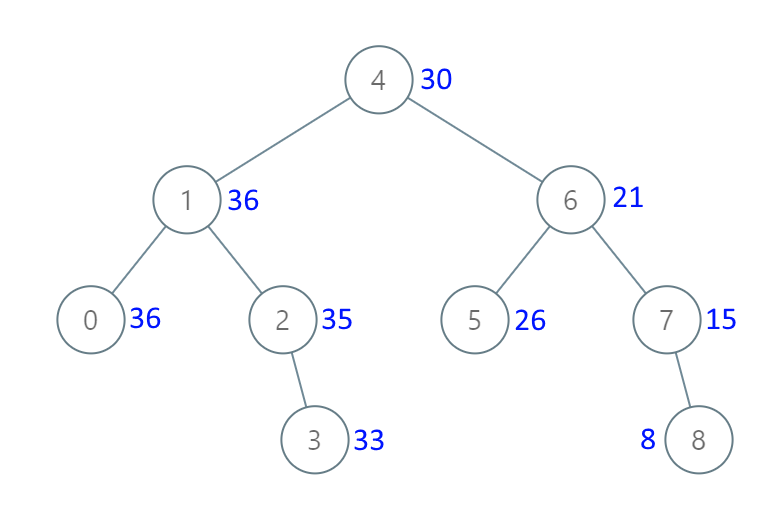# 538. Convert BST to Greater Tree

Medium
Given the `root` of a Binary Search Tree (BST), convert it to a Greater Tree such that every key of the original BST is changed to the original key plus the sum of all keys greater than the original key in BST.
As a reminder, a binary search tree is a tree that satisfies these constraints:
• The left subtree of a node contains only nodes with keys less than the node's key.
• The right subtree of a node contains only nodes with keys greater than the node's key.
• Both the left and right subtrees must also be binary search trees.
Example 1:Input: root = [4,1,6,0,2,5,7,null,null,null,3,null,null,null,8]
Output: [30,36,21,36,35,26,15,null,null,null,33,null,null,null,8]
Example 2:
Input: root = [0,null,1]
Output: [1,null,1]
Constraints:
• The number of nodes in the tree is in the range `[0, 10^4]`.
• `-10^4 <= Node.val <= 10^4`
• All the values in the tree are unique.
• `root` is guaranteed to be a valid binary search tree.

### 解題

Runtime: 9 ms, faster than 100%
Memory Usage: 6.7 MB, less than 79.7%
/**
* Definition for a binary tree node.
* type TreeNode struct {
* Val int
* Left *TreeNode
* Right *TreeNode
* }
*/
func convertBST(root *TreeNode) *TreeNode {
sum := 0
var helper func(*TreeNode)
helper = func(root *TreeNode) {
if root == nil { return }
helper(root.Right)
sum += root.Val
root.Val = sum
helper(root.Left)
}
helper(root)
return root
}